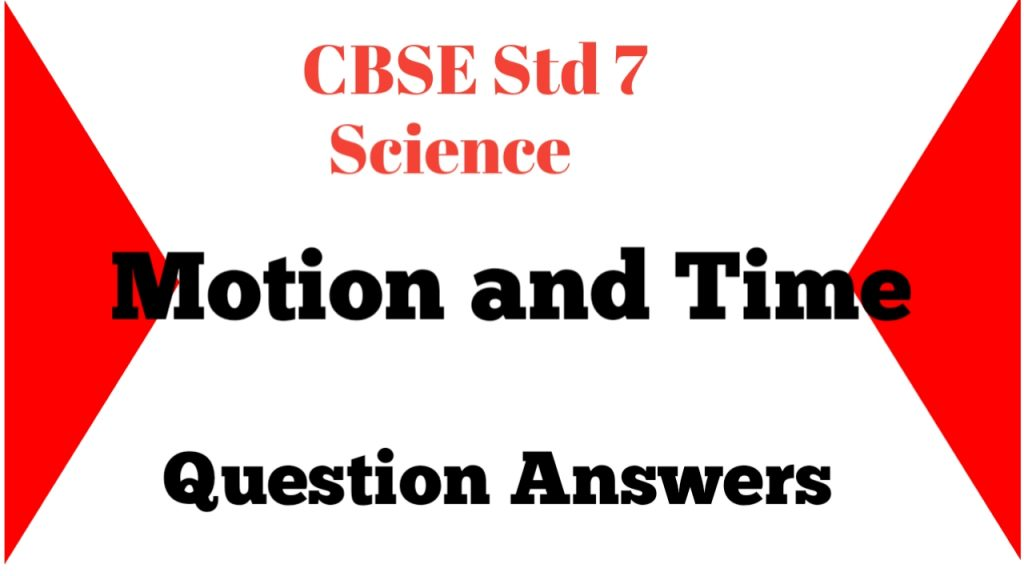# Motion and Time

## Exercise

1). Classify the following as motion along a straight line, circular or oscillatory motion:

(i) Motion of your hands while running.

(ii) Motion of a horse pulling a cart on a straight road.

(iii) Motion of a child in a merry-go-round.

(iv) Motion of a child on a see-saw.

(v) Motion of the hammer of an electric bell.

(vi) Motion of a train on a straight bridge.

(i) Motion of your hands while running.

Ans: oscillatory motion

(ii) Motion of a horse pulling a cart on a straight road.

Ans: Straight line

(iii) Motion of a child in a merry-go-round.

Ans: Circular motion

(iv) Motion of a child on a see-saw.

Ans: Oscillatory motion

(v) Motion of the hammer of an electric bell.

Ans: Oscillatory motion.

(vi) Motion of a train on a straight bridge.

Ans: Straight line.

2). Which of the following are not correct?

(i) The basic unit of time is second.

Ans: Correct.

(ii) Every object moves with a constant speed.

Ans: Incorrect.

(iii) Distances between two cities are measured in kilometres.

Ans: Correct.

(iv) The time period of a given pendulum is constant.

Ans: Incorrect.

(v) The speed of a train is expressed in m/h.

Ans: Incorrect.

3). A simple pendulum takes 32 s to complete 20 oscillations. What is the time period of the pendulum?

Given: No. of oscillations = 20

Time taken = 32 sec

To find: Time Period= ?

Time period = time taken/no. of oscillations

= 32/20

= 16/10

= 1.6

Ans: Time period of the pendulum = 1.6 sec

4). The distance between two stations is 240 km. A train takes 4 hours to cover this distance. Calculate the speed of the train.

Given: Distance = 240 km

Time = 4 hours

To find: Speed of the train = ?

Speed = distance / time

= 240/4

= 60 km/hr.

Ans: Speed of the train = 60 km/hr.

5). The odometer of a car reads 57321.0 km when the clock shows the time 08:30 AM. What is the distance moved by the car, if at 08:50 AM, the odometer reading has changed to 57336.0 km? Calculate the speed of the car in km/min during this time. Express the speed in km/h also.

Distance travelled by the car = 57336.0 – 57321. 0

= 15 km

= 15000m

Time = 08: 50 – 08:30 = 20 min

Speed = distance/time

= 15000 / 20

= 1500/2

= 750 m/min

= 750 X 60/1000

= 45000/1000

= 45 km/hr

Ans: The distance moved by the car = 15 km

Speed of the car = 45 km/hr

6). Salma takes 15 minutes from her house to reach her school on a bicycle. If the bicycle has a speed of 2 m/s, calculate the distance between her house and the school.

Given: Time = 15 min = 15 X 60 = 900 sec

Speed = 2 m/s

To find: Distance = ?

Distance = speed X time

= 2 X 900

= 1800 m

Ans: The distance between Salma’s house and the school = 1800 m.

7). Show the shape of the distance-time graph for the motion in the following cases:

(i) A car moving with a constant speed.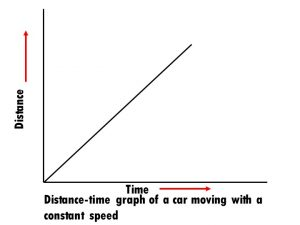(ii) A car parked on a side road.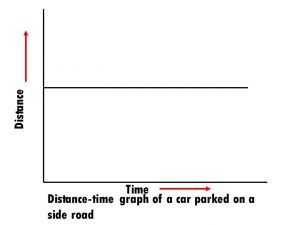8). Which of the following relations is correct?

(i) Speed = Distance × Time        (ii) Speed = Distance/Time

(iii) Speed = Time /Distance       (iv) Speed = Distance × Time

Ans: Distance/time

9). The basic unit of speed is:

(i) km/min                                       (ii) m/min

(iii) km/h                                         (iv) m/s

Ans: m/s

10). A car moves with a speed of 40 km/h for 15 minutes and then with a speed of 60 km/h for the next 15 minutes. The total distance covered by the car is:

(i) 100 km                                        (ii) 25 km

(iii) 15 km                                        (iv) 10 km

Ans: speed = 40 km/h

Time = 15 min = 15/60 hr = ¼ hr

Distance = speed X time

= 40 X ¼

= 10 km

speed = 60 km/h

Time = 15 min = 15/60 hr = ¼ hr

Distance = speed X time

= 60 X ¼

= 15 km

Total distance = 10 + 15

= 25 km

(*) 11). Suppose the two photographs, shown in Fig. 13.1 and Fig. 13.2, had been taken at an interval of 10 seconds. If a distance of 100 metres is shown by 1 cm in these photographs, calculate the speed of the fastest car.

Do yourself.

12). Fig. 13.15 shows the distance-time graph for the motion of two vehicles A and B. Which one of them is moving faster?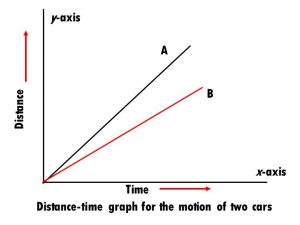Ans: A is moving faster.

13). Which of the following distance-time graphs shows a truck moving with speed which is not constant?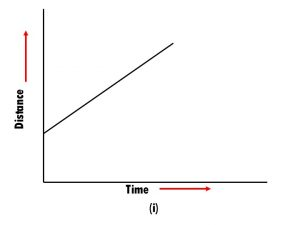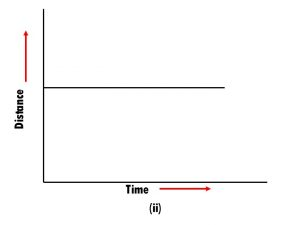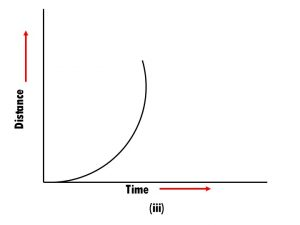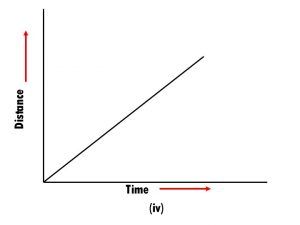Ans: (ii) and (iii).

Fill in the blanks.

(i). The metallic ball is called the bob of the pendulum.

(ii). The to and fro motion of a simple pendulum is an example of a periodic or an oscillatory motion.

(iii). The basic unit of time is second.

(iv). The basic unit of speed is m/s.

(v).  The distance-time graph for the motion of an object moving with a constant speed is a straight line.

True or false.

(i). The distance moved by objects in a given interval of time can help us to decide which one is faster or slower.

Ans: True.

(ii). Car seldom moves with a constant speed.

Ans: True.

(iii). In case of uniform motion, the average speed is same as the actual speed.

Ans: True.

(iv). The basic unit of time is minutes.

Ans: False

(v) A pendulum of a given length takes always the same time to complete one oscillations.

Ans: True.

(vi). The symbols of all units are written in singular.

Ans: True.

Define.

(i). Speed

Ans: Distance covered by an object in a unit time is called as speed of that object.

(ii). Uniform motion

Ans: If an object is moving along a straight line with a constant speed it is said to be in uniform motion.

(iii). Non-uniform motion

Ans: If the speed of an object moving along a straight line keeps changing, its motion is said to be non-uniform.

(iv). Time period

Ans: The time taken by the pendulum to complete one oscillations is called its time period.

1). What is a simple pendulum?

Ans: A simple pendulum consitsts of a small metallic ball or a piece of stone suspended from a rigid stand by a thread.

2) What are quartz clocks?

Ans: Clocks or watches that have an electric circuit with one or more cells are called as quartz clocks.

3). What is the advantage of using quartz clocks?

Ans: The time measured by quartz clocks is much more accurate than that by the earlier clocks.

4). What are x-axis and y-axis in a graph?

Ans: The horizontal line in a graph is called as x-axis and vertical line is called as y-axis.

5). What is origin in a graph?

Ans: The intersection of x-axis and y-axis is called origin.

1). Nutrition in Plants

2). Nutrition in Animals

3). Fibre to Fabric

4). Heat

5). Acids, Bases and Salts

6). Physical and Chemical Changes

7). Weather, Climate and Adaptations of Animals to Climate

8). Winds, Storms and Cyclones

9). Soil

10). Respiration in Organisms

11). Transportation in Animals and Plants

12). Reproduction in Plants

13). Motion and Time

14). Electric Currents and its Effects

15). Light

16). Water: A Precious Resource

17). Forests: Our Lifeline

18). Wastewater Storyerror: Content is protected !!